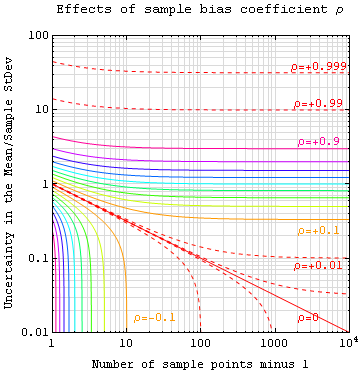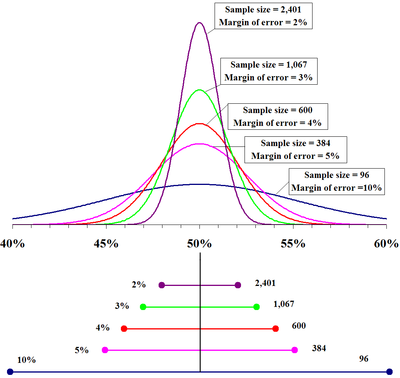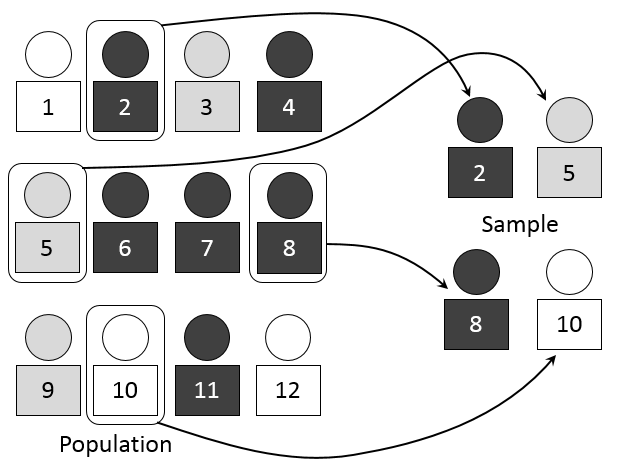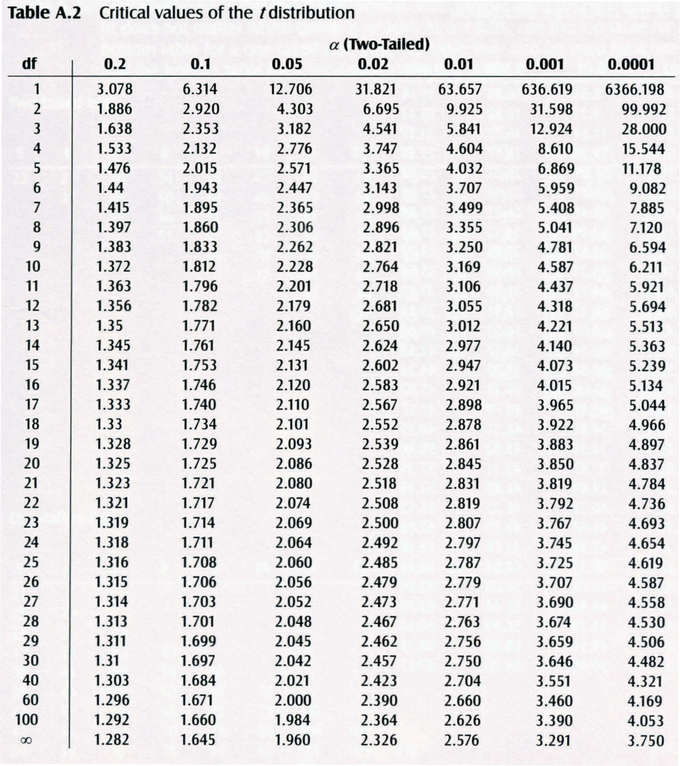## Estimation

Estimating population parameters from sample parameters is one of the major applications of inferential statistics.

### Learning Objectives

Describe how to estimate population parameters with consideration of error

### Key Takeaways

#### Key Points

• Seldom is the sample statistic exactly equal to the population parameter, so a range of likely values, or an estimate interval, is often given.
• Error is defined as the difference between the population parameter and the sample statistics.
• Bias (or systematic error ) leads to a sample mean that is either lower or higher than the true mean.
• Mean-squared error is used to indicate how far, on average, the collection of estimates are from the parameter being estimated.
• Mean-squared error is used to indicate how far, on average, the collection of estimates are from the parameter being estimated.

#### Key Terms

• interval estimate: A range of values used to estimate a population parameter.
• error: The difference between the population parameter and the calculated sample statistics.
• point estimate: a single value estimate for a population parameter

One of the major applications of statistics is estimating population parameters from sample statistics. For example, a poll may seek to estimate the proportion of adult residents of a city that support a proposition to build a new sports stadium. Out of a random sample of 200 people, 106 say they support the proposition. Thus in the sample, 0.53 ($\frac{106}{200}$) of the people supported the proposition. This value of 0.53 (or 53%) is called a point estimate of the population proportion. It is called a point estimate because the estimate consists of a single value or point.

It is rare that the actual population parameter would equal the sample statistic. In our example, it is unlikely that, if we polled the entire adult population of the city, exactly 53% of the population would be in favor of the proposition. Instead, we use confidence intervals to provide a range of likely values for the parameter.

For this reason, point estimates are usually supplemented by interval estimates or confidence intervals. Confidence intervals are intervals constructed using a method that contains the population parameter a specified proportion of the time. For example, if the pollster used a method that contains the parameter 95% of the time it is used, he or she would arrive at the following 95% confidence interval: $0.46 < \text{p} <0.60$. The pollster would then conclude that somewhere between 46% and 60% of the population supports the proposal. The media usually reports this type of result by saying that 53% favor the proposition with a margin of error of 7%.

### Error and Bias

Assume that $\theta$ (the Greek letter “theta”) is the value of the population parameter we are interested in. In statistics, we would represent the estimate as $\hat { \theta }$ (read theta-hat). We know that the estimate $\hat { \theta }$ would rarely equal the actual population parameter $\theta$. There is some level of error associated with it. We define this error as $e\left( \text{x} \right) =\hat { \theta } \left( \text{x} \right) -\theta$.

All measurements have some error associated with them. Random errors occur in all data sets and are sometimes known as non-systematic errors. Random errors can arise from estimation of data values, imprecision of instruments, etc. For example, if you are reading lengths off a ruler, random errors will arise in each measurement as a result of estimating between which two lines the length lies. Bias is sometimes known as systematic error. Bias in a data set occurs when a value is consistently under or overestimated. Bias can also arise from forgetting to take into account a correction factor or from instruments that are not properly calibrated. Bias leads to a sample mean that is either lower or higher than the true mean.Sample Bias Coefficient: An estimate of expected error in the sample mean of variable $\text{A}$, sampled at $\text{N}$ locations in a parameter space $\text{x}$, can be expressed in terms of sample bias coefficient $\rho$ — defined as the average auto-correlation coefficient over all sample point pairs. This generalized error in the mean is the square root of the sample variance (treated as a population) times $\frac{1+(\text{N}-1)\rho}{(\text{N}-1)(1-\rho)}$. The $\rho = 0$ line is the more familiar standard error in the mean for samples that are uncorrelated.

### Mean-Squared Error

The mean squared error (MSE) of $\hat { \theta }$ is defined as the expected value of the squared errors. It is used to indicate how far, on average, the collection of estimates are from the single parameter being estimated $\left( \theta \right)$. Suppose the parameter is the bull’s-eye of a target, the estimator is the process of shooting arrows at the target, and the individual arrows are estimates (samples). In this case, high MSE means the average distance of the arrows from the bull’s-eye is high, and low MSE means the average distance from the bull’s-eye is low. The arrows may or may not be clustered. For example, even if all arrows hit the same point, yet grossly miss the target, the MSE is still relatively large. However, if the MSE is relatively low, then the arrows are likely more highly clustered (than highly dispersed).

## Estimates and Sample Size

Here, we present how to calculate the minimum sample size needed to estimate a population mean ($\mu$) and population proportion ($\text{p}$).

### Learning Objectives

Calculate sample size required to estimate the population mean

### Key Takeaways

#### Key Points

• Before beginning a study, it is important to determine the minimum sample size, taking into consideration the desired level of confidence, the margin of error, and a previously observed sample standard deviation.
• When $\text{n} \geq 30$, the sample standard deviation ($\text{s}$) can be used in place of the population standard deviation ($\sigma$).
• The minimum sample size $\text{n}$ needed to estimate the population mean ($\mu$) is calculated using the formula: $n={ \left( \frac { { \text{Z} }_{ \frac { \alpha }{ 2 } }\sigma }{ \text{E} } \right) }^{ 2 }$.${ \left( \frac { { \text{Z} }_{ \frac { \alpha }{ 2 } }\sigma }{ \text{E} } \right) }^{ 2 }$.
• The minimum sample size $\text{n}$ needed to estimate the population proportion ($\text{p}$) is calculated using the formula: $\text{n}=\text{p}'\text{q}'\left( \frac { { \text{Z} }_{ \frac { \alpha }{ 2 } } }{ \text{E} } \right) ^{ 2 }$.

#### Key Terms

• margin of error: An expression of the lack of precision in the results obtained from a sample.

### Determining Sample Size Required to Estimate the Population Mean ($\mu$)

Before calculating a point estimate and creating a confidence interval, a sample must be taken. Often, the number of data values needed in a sample to obtain a particular level of confidence within a given error needs to be determined before taking the sample. If the sample is too small, the result may not be useful, and if the sample is too big, both time and money are wasted in the sampling. The following text discusses how to determine the minimum sample size needed to make an estimate given the desired confidence level and the observed standard deviation.

First, consider the margin of error, $\text{E}$, the greatest possible distance between the point estimate and the value of the parameter it is estimating. To calculate $\text{E}$, we need to know the desired confidence level (${ \text{Z} }_{ \frac { \alpha }{ 2 } }$) and the population standard deviation, $\sigma$. When $\text{n} \geq 30$, the sample standard deviation ($\text{s}$) can be used to approximate the population standard deviation $\sigma$.

$\displaystyle \text{E}={ \text{Z} }_{ \frac { \alpha }{ 2 } }\frac { \sigma }{ \sqrt { \text{n} } }$

To change the size of the error ($\text{E}$), two variables in the formula could be changed: the level of confidence (${ \text{Z} }_{ \frac { \alpha }{ 2 } }$) or the sample size ($\text{n}$). The standard deviation ($\sigma$) is a given and cannot change.

As the confidence increases, the margin of error ($\text{E}$) increases. To ensure that the margin of error is small, the confidence level would have to decrease. Hence, changing the confidence to lower the error is not a practical solution.

As the sample size ($\text{n}$) increases, the margin of error decreases. The question now becomes: how large a sample is needed for a particular error? To determine this, begin by solving the equation for the $\text{E}$ in terms of $\text{n}$:Sample size compared to margin of error: The top portion of this graphic depicts probability densities that show the relative likelihood that the “true” percentage is in a particular area given a reported percentage of 50%. The bottom portion shows the 95% confidence intervals (horizontal line segments), the corresponding margins of error (on the left), and sample sizes (on the right). In other words, for each sample size, one is 95% confident that the “true” percentage is in the region indicated by the corresponding segment. The larger the sample is, the smaller the margin of error is.

$\text{n}={ \left( \frac { { \text{Z} }_{ \frac { \alpha }{ 2 } }\sigma }{ \text{E} } \right) }^{ 2 }$

where ${ \text{Z} }_{ \frac { \alpha }{ 2 } }$ is the critical $\text{z}$ score based on the desired confidence level, $\text{E}$ is the desired margin of error, and $\sigma$ is the population standard deviation.

Since the population standard deviation is often unknown, the sample standard deviation from a previous sample of size $\text{n}\geq 30$ may be used as an approximation to $\text{s}$. Now, we can solve for $\text{n}$ to see what would be an appropriate sample size to achieve our goals. Note that the value found by using the formula for sample size is generally not a whole number. Since the sample size must be a whole number, always round up to the next larger whole number.

### Example

Suppose the scores on a statistics final are normally distributed with a standard deviation of 10 points. Construct a 95% confidence interval with an error of no more than 2 points.

#### Solution

$\text{Z}_{0.025} = 1.645$

$\text{E}=2$

$\sigma = 10$

$\text{n}={ \left( \frac { 1.645\left( 10 \right) }{ 2 } \right) }^{ 2 }=8.225^2 = 67.75$

So, a sample of size of 68 must be taken to create a 95% confidence interval with an error of no more than 2 points.

### Determining Sample Size Required to Estimate Population Proportion ($\text{p}$)

The calculations for determining sample size to estimate a proportion ($\text{p}$) are similar to those for estimating a mean ($\mu$). In this case, the margin of error, $\text{E}$, is found using the formula:

$\text{E}={ \text{Z} }_{ \frac { \alpha }{ 2 } }\sqrt { \frac { \text{p}'\text{q}' }{ \text{n} } }$

where:

• $\text{p}' = \frac{\text{x}}{\text{n}}$ is the point estimate for the population proportion
• $\text{x}$ is the number of successes in the sample
• $\text{n}$ is the number in the sample; and
• $\text{q}' = 1-\text{p}'$

Then, solving for the minimum sample size $\text{n}$ needed to estimate $\text{p}$:

$\text{n}=\text{p}'\text{q}'\left( \frac { { \text{Z} }_{ \frac { \alpha }{ 2 } } }{ \text{E} } \right) ^{ 2 }$

### Example

The Mesa College mathematics department has noticed that a number of students place in a non-transfer level course and only need a 6 week refresher rather than an entire semester long course. If it is thought that about 10% of the students fall in this category, how many must the department survey if they wish to be 95% certain that the true population proportion is within $\pm 5\%$?

#### Solution

$\text{Z}=1.96 \\ \text{E}=0.05 \\ \text{p}' = 0.1 \\ \text{q}' = 0.9 \\ \text{n}=\left( 0.1 \right) \left( 0.9 \right) \left( \frac { 1.96 }{ 0.05 } \right) ^{ 2 }\approx 138.3$

So, a sample of size of 139 must be taken to create a 95% confidence interval with an error of $\pm 5\%$.

## Estimating the Target Parameter: Point Estimation

Point estimation involves the use of sample data to calculate a single value which serves as the “best estimate” of an unknown population parameter.

### Learning Objectives

Contrast why MLE and linear least squares are popular methods for estimating parameters

### Key Takeaways

#### Key Points

• In inferential statistics, data from a sample is used to “estimate” or “guess” information about the data from a population.
• The most unbiased point estimate of a population mean is the sample mean.
• Maximum-likelihood estimation uses the mean and variance as parameters and finds parametric values that make the observed results the most probable.
• Linear least squares is an approach fitting a statistical model to data in cases where the desired value provided by the model for any data point is expressed linearly in terms of the unknown parameters of the model (as in regression ).

#### Key Terms

• point estimate: a single value estimate for a population parameter

In inferential statistics, data from a sample is used to “estimate” or “guess” information about the data from a population. Point estimation involves the use of sample data to calculate a single value or point (known as a statistic) which serves as the “best estimate” of an unknown population parameter. The point estimate of the mean is a single value estimate for a population parameter. The most unbiased point estimate of a population mean (µ) is the sample mean ($\bar { \text{x} }$).Simple random sampling of a population: We use point estimators, such as the sample mean, to estimate or guess information about the data from a population. This image visually represents the process of selecting random number-assigned members of a larger group of people to represent that larger group.

### Maximum Likelihood

A popular method of estimating the parameters of a statistical model is maximum-likelihood estimation (MLE). When applied to a data set and given a statistical model, maximum-likelihood estimation provides estimates for the model’s parameters. The method of maximum likelihood corresponds to many well-known estimation methods in statistics. For example, one may be interested in the heights of adult female penguins, but be unable to measure the height of every single penguin in a population due to cost or time constraints. Assuming that the heights are normally (Gaussian) distributed with some unknown mean and variance, the mean and variance can be estimated with MLE while only knowing the heights of some sample of the overall population. MLE would accomplish this by taking the mean and variance as parameters and finding particular parametric values that make the observed results the most probable, given the model.

In general, for a fixed set of data and underlying statistical model, the method of maximum likelihood selects the set of values of the model parameters that maximizes the likelihood function. Maximum-likelihood estimation gives a unified approach to estimation, which is well-defined in the case of the normal distribution and many other problems. However, in some complicated problems, maximum-likelihood estimators are unsuitable or do not exist.

### Linear Least Squares

Another popular estimation approach is the linear least squares method. Linear least squares is an approach fitting a statistical model to data in cases where the desired value provided by the model for any data point is expressed linearly in terms of the unknown parameters of the model (as in regression). The resulting fitted model can be used to summarize the data, to estimate unobserved values from the same system, and to understand the mechanisms that may underlie the system.

Mathematically, linear least squares is the problem of approximately solving an over-determined system of linear equations, where the best approximation is defined as that which minimizes the sum of squared differences between the data values and their corresponding modeled values. The approach is called “linear” least squares since the assumed function is linear in the parameters to be estimated. In statistics, linear least squares problems correspond to a statistical model called linear regression which arises as a particular form of regression analysis. One basic form of such a model is an ordinary least squares model.

## Estimating the Target Parameter: Interval Estimation

Interval estimation is the use of sample data to calculate an interval of possible (or probable) values of an unknown population parameter.

### Learning Objectives

Use sample data to calculate interval estimation

### Key Takeaways

#### Key Points

• The most prevalent forms of interval estimation are confidence intervals (a frequentist method) and credible intervals (a Bayesian method).
• When estimating parameters of a population, we must verify that the sample is random, that data from the population have a Normal distribution with mean $\mu$ and standard deviation $\sigma$, and that individual observations are independent.
• In order to specify a specific $\text{t}$-distribution, which is different for each sample size $\text{n}$, we use its degrees of freedom, which is denoted by $\text{df}$, and $\text{df} = \text{n}-1$.
• If we wanted to calculate a confidence interval for the population mean, we would use: $\bar{\text{x}}\pm \text{t}^{*}\frac{\text{s}}{\sqrt{\text{n}}}$, where $\text{t}^*$ is the critical value for the $\text{t}(\text{n}-1)$ distribution.

#### Key Terms

• t-distribution: a family of continuous probability disrtibutions that arises when estimating the mean of a normally distributed population in situations where the sample size is small and population standard devition is unknown
• critical value: the value corresponding to a given significance level

Interval estimation is the use of sample data to calculate an interval of possible (or probable) values of an unknown population parameter. The most prevalent forms of interval estimation are:

• confidence intervals (a frequentist method); and
• credible intervals (a Bayesian method).

Other common approaches to interval estimation are:

• Tolerance intervals
• Prediction intervals – used mainly in Regression Analysis
• Likelihood intervals

### Example: Estimating the Population Mean

How can we construct a confidence interval for an unknown population mean $\mu$ when we don’t know the population standard deviation $\sigma$? We need to estimate from the data in order to do this. We also need to verify three conditions about the data:

1. The data is from a simple random sample of size $\text{n}$ from the population of interest.
2. Data from the population have a Normal distribution with mean and standard deviation. These are both unknown parameters.
3. The method for calculating a confidence interval assumes that individual observations are independent.

The sample mean $\bar{\text{x}}$ has a Normal distribution with mean and standard deviation $\frac{\sigma }{\sqrt{\text{n}}}$. Since we don’t know $\sigma$, we estimate it using the sample standard deviation $\text{s}$. So, we estimate the standard deviation of $\bar{\text{x}}$ using $\frac{\text{s} }{\sqrt{\text{n}}}$, which is called the standard error of the sample mean.

### The $\text{t}$-Distribution

When we do not know $\frac{\sigma}{\sqrt{\text{n}}}$, we use $\frac{\text{s} }{\sqrt{\text{n}}}$. The distribution of the resulting statistic, $\text{t}$, is not Normal and fits the $\text{t}$-distribution. There is a different $\text{t}$-distribution for each sample size $\text{n}$. In order to specify a specific $\text{t}$-distribution, we use its degrees of freedom, which is denoted by $\text{df}$, and $\text{df}= \text{n}-1$.$\text{t}$-Distribution: A plot of the $\text{t}$-distribution for several different degrees of freedom.

If we wanted to estimate the population mean, we can now put together everything we’ve learned. First, draw a simple random sample from a population with an unknown mean. A confidence interval for is calculated by: $\bar{\text{x}}\pm \text{t}^{*}\frac{\text{s}}{\sqrt{\text{n}}}$, where $\text{t}^*$ is the critical value for the $\text{t}(\text{n}-1)$ distribution.$\text{t}$-Table: Critical values of the $\text{t}$-distribution.

## Estimating a Population Proportion

In order to estimate a population proportion of some attribute, it is helpful to rely on the proportions observed within a sample of the population.

### Learning Objectives

Derive the population proportion using confidence intervals

### Key Takeaways

#### Key Points

• If you want to rely on a sample, it is important that the sample be random (i.e., done in such as way that each member of the underlying population had an equal chance of being selected for the sample).
• As the size of a random sample increases, there is greater “confidence” that the observed sample proportion will be “close” to the actual population proportion.
• For general estimates of a population proportion, we use the formula: $\sqrt{\frac{\hat{\text{p}}(1-\hat{\text{p}})}{\text{n}}}$.
• To estimate a population proportion to be within a specific confidence interval, we use the formula: $\hat{\text{p}}\pm \text{z}^{*}\sqrt{\frac{\hat{\text{p}}(1-\hat{\text{p}})}{\text{n}}}$.

#### Key Terms

• confidence interval: A type of interval estimate of a population parameter used to indicate the reliability of an estimate.
• standard error: A measure of how spread out data values are around the mean, defined as the square root of the variance.

You do not need to be a math major or a professional statistician to have an intuitive appreciation of the following:

• In order to estimate the proportions of some attribute within a population, it would be helpful if you could rely on the proportions observed within a sample of the population.
• If you want to rely on a sample, it is important that the sample be random. This means that the sampling was done in such a way that each member of the underlying population had an equal chance of being selected for the sample.
• The size of the sample is important. As the size of a random sample increases, there is greater “confidence” that the observed sample proportion will be “close” to the actual population proportion. If you were to toss a fair coin ten times, it would not be that surprising to get only 3 or fewer heads (a sample proportion of 30% or less). But if there were 1,000 tosses, most people would agree – based on intuition and general experience – that it would be very unlikely to get only 300 or fewer heads. In other words, with the larger sample size, it is generally apparent that the sample proportion will be closer to the actual “population” proportion of 50%.
• While the sample proportion might be the best estimate of the total population proportion, you would not be very confident that this is exactly the population proportion.

### Finding the Population Proportion Using Confidence Intervals

Let’s look at the following example. Assume a political pollster samples 400 voters and finds 208 for Candidate $\text{A}$ and 192 for Candidate $\text{B}$. This leads to an estimate of 52% as $\text{A}$‘s support in the population. However, it is unlikely that $\text{A}$‘s support actual will be exactly 52%. We will call 0.52 $\hat{\text{p}}$ (pronounced “p-hat”). The population proportion, $\text{p}$, is estimated using the sample proportion $\hat{\text{p}}$. However, the estimate is usually off by what is called the standard error (SE). The SE can be calculated by:

$\displaystyle \sqrt{\frac{\hat{\text{p}}(1-\hat{\text{p}})}{\text{n}}}$

where $\text{n}$ is the sample size. So, in this case, the SE is approximately equal to 0.02498. Therefore, a good population proportion for this example would be $0.52 \pm 0.2498$.

Often, statisticians like to use specific confidence intervals for $\text{p}$. This is computed slightly differently, using the formula:

$\displaystyle \hat{\text{p}}\pm \text{z}^{*}\sqrt{\frac{\hat{\text{p}}(1-\hat{\text{p}})}{\text{n}}}$

where $\text{z}^*$ is the upper critical value of the standard normal distribution. In the above example, if we wished to calculate $\text{p}$ with a confidence of 95%, we would use a $\text{Z}$-value of 1.960 (found using a critical value table), and we would find $\text{p}$ to be estimated as $0.52\pm0.04896$. So, we could say with 95% confidence that between 47.104% and 56.896% of the people will vote for candidate $\text{A}$.Critical Value Table: $\text{t}$-table used for finding $\text{z}^*$ for a certain level of confidence.

A simple guideline – If you use a confidence level of $\text{X}\%$, you should expect $(100-\text{X})\%$ of your conclusions to be incorrect. So, if you use a confidence level of 95%, you should expect 5% of your conclusions to be incorrect.